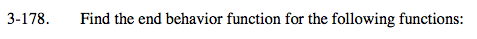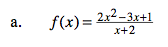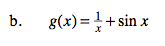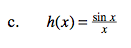### Home > CALC > Chapter Ch3 > Lesson 3.4.3 > Problem3-178

3-178.End behavior describes the shape of the function if we ignore all vertical asymptotes and holes.

If the function has a horizontal asymotote, then that is its end behavior.
If a function has a slant asymptote, then that is it's end behavior.

If a function oscillates as x →∞ or x →−∞, then it has no end behavior.For rational functions, end behavior can often be found using polynomial division (and ignoring the remainder).Use your calculator to sketch a graph of g(x). What does it look like as x →∞ and x →−∞?Examine.

Compare the numerator and denominator. Which has the highest power term as x → ∞? (Remember that y = sinx never gets higher than 1 or lower than −1.) What does that say about asymptotes?